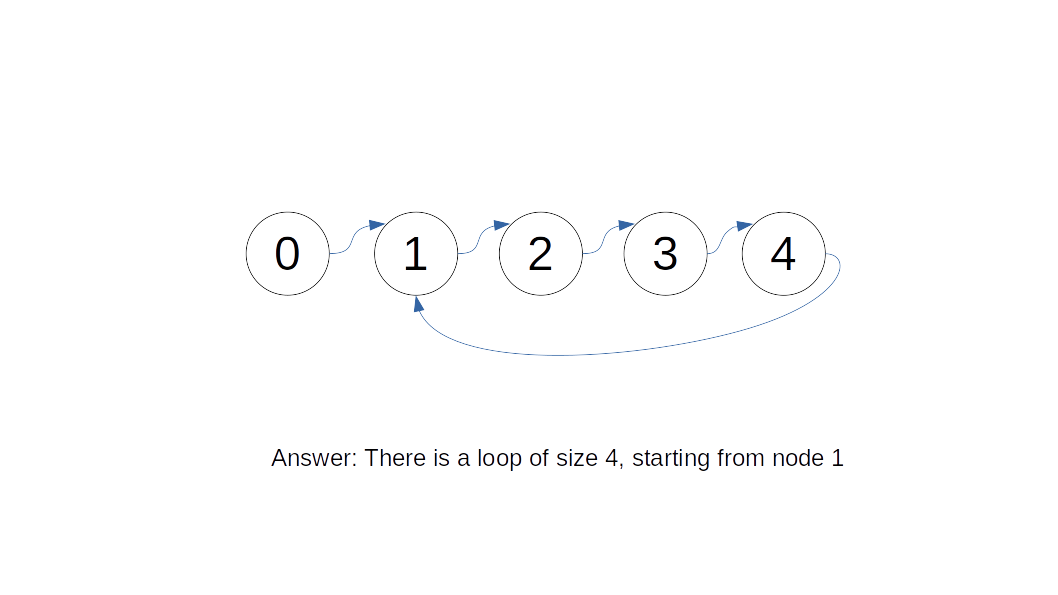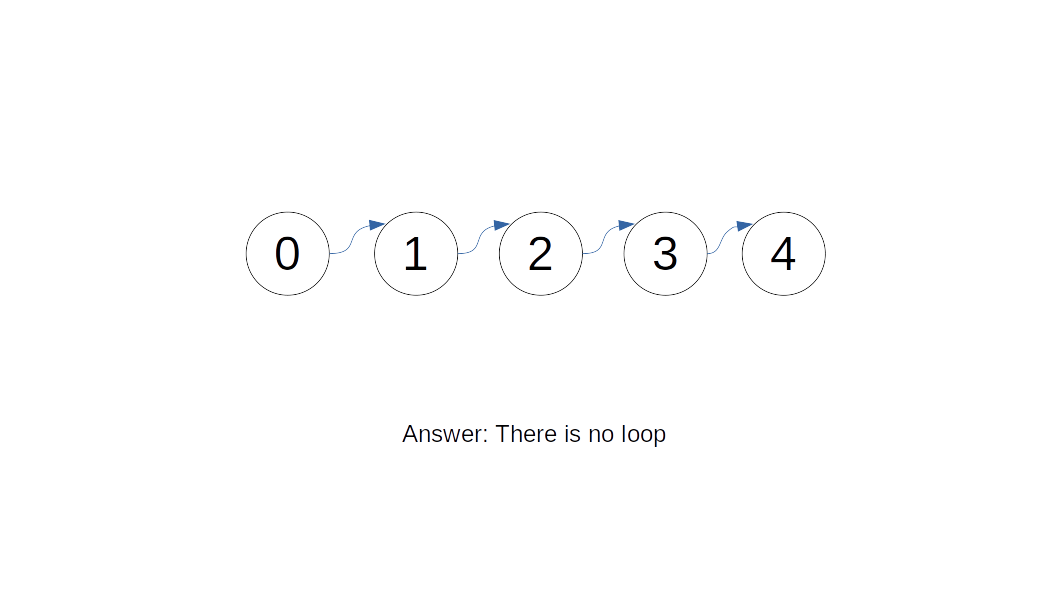Given a linked list with potentially a loop, determine whether the linked list from the first node contains a cycle in it. For bonus points, do this with constant space.

#### Parameters

• `nodes`: The first node of a linked list with potentially a loop.

#### Result

• Whether there is a loop contained in the linked list.

### Examples

#### Example 1

Input:Output:

`true`

#### Example 2

Input:Output:

`false`

### Constraints

• `1 <= len(nodes) <= 10^5`

## Solution

If a linked list has no loop, then when we iterate through the list, we will eventually reach the end of the list, at which point we can simply return. However, the challenge is figuring out how to terminate the program if it finds a loop. Otherwise the program would go on forever.

A simple solution would be to use a set to store the information. We store all the nodes we have been through and check if we have been there each time we move. However, a set is not constant memory, and there might be issues with hashing and whatnot. Clearly there is a better way.

Introducing Floyd's Cycle Finding Algorithm, also known as the Tortoise and Hare Algorithm. The idea is to have two pointers, the fast pointer (or "hare") moves at double speed of the slow pointer (or "tortoise"). Each cycle, the tortoise moves once and the hare moves twice. The idea is that since the cycle must have integer size, when the tortoise and the hare are both in the cycle, their distance difference must also be an integer. Then, each cycle, because of their speed difference, the distance between them constantly reduces until they meet, in which case we know there is a cycle. However, if there is no cycle, they will never meet because the speed of the hare is always faster.

Time Complexity: `O(n)`

Technically `O(n/2)` but again we factor out the constant and we are left with linear time. Worst case we have, `O(2n)` as the small pointer moves around the entire array once. Either way we have `O(n)` time complexity.

This is a graphics that explains the idea:

Below is an implementation.

``````1class Node:
2    def __init__(self, val, next=None):
3        self.val = val
4        self.next = next
5
6def node_next(node):
7    return node.next or node
8
9def has_cycle(nodes: Node) -> bool:
10    tortoise = node_next(nodes)
11    hare = node_next(node_next(nodes))
12    while tortoise != hare and hare.next is not None:
13        tortoise = node_next(tortoise)
14        hare = node_next(node_next(hare))
15    return hare.next is not None
16
17if __name__ == '__main__':
18    raw_input = [int(x) for x in input().split()]
19    nodes_list = []
20    for i, entry in enumerate(raw_input):
21        nodes_list.append(Node(i))
22    for i, entry in enumerate(raw_input):
23        if entry != -1:
24            nodes_list[i].next = nodes_list[entry]
25    nodes = nodes_list
26    res = has_cycle(nodes)
27    print('true' if res else 'false')
28``````